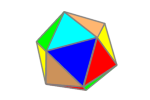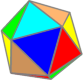# IcosahedronThe icosahedron has 12 equilateral triangles as faces.

It can be split into 3 parts, a pentagonal anti-prism and two pentagonal pyramids. We will start by looking at a pentagonal pyramid.

\begin{align}\cos \angle ACB &= \frac{1^2 + S^2 – H^2}{2\cdot1\cdot S}\\ &= \frac{1^2 + \frac34 – \frac{5+2\sqrt5}{4}}{2\cdot 1\cdot \frac{\sqrt3}{2}}\\ &= \frac{\frac{2-2\sqrt5}{4} }{\sqrt3} \\ &= \frac{2-2\sqrt5}{4\sqrt3} \\ &= \frac{1-\sqrt5}{2\sqrt3}\\ACB &= 110.905157\ldots^\circ \end{align}
\begin{align}Icosahedron\ Dihedral \angle &= \cos^{-1}\Bigg(\frac{S^2 + S^2 – \Phi^2}{2\cdot S\cdot S}\Bigg)\\ &=\cos^{-1}\Bigg(\frac{ \frac34 + \frac34 – \frac{6+2\sqrt5}{4} }{ 2\cdot \frac{\sqrt3}{2} \cdot \frac{\sqrt3}{2} }\Bigg)\\ &=\cos^{-1}\Bigg(\frac{ – \frac{2\sqrt5}{4} }{ 2\cdot \frac34 }\Bigg)\\ &=\cos^{-1}\Bigg(\frac{ – 2\sqrt5 }{ 2\cdot 3 }\Bigg)\\ &=\cos^{-1}\Bigg(\frac{-\sqrt5}{3}\Bigg)\\ &= 138.1896851\ldots^\circ \end{align}